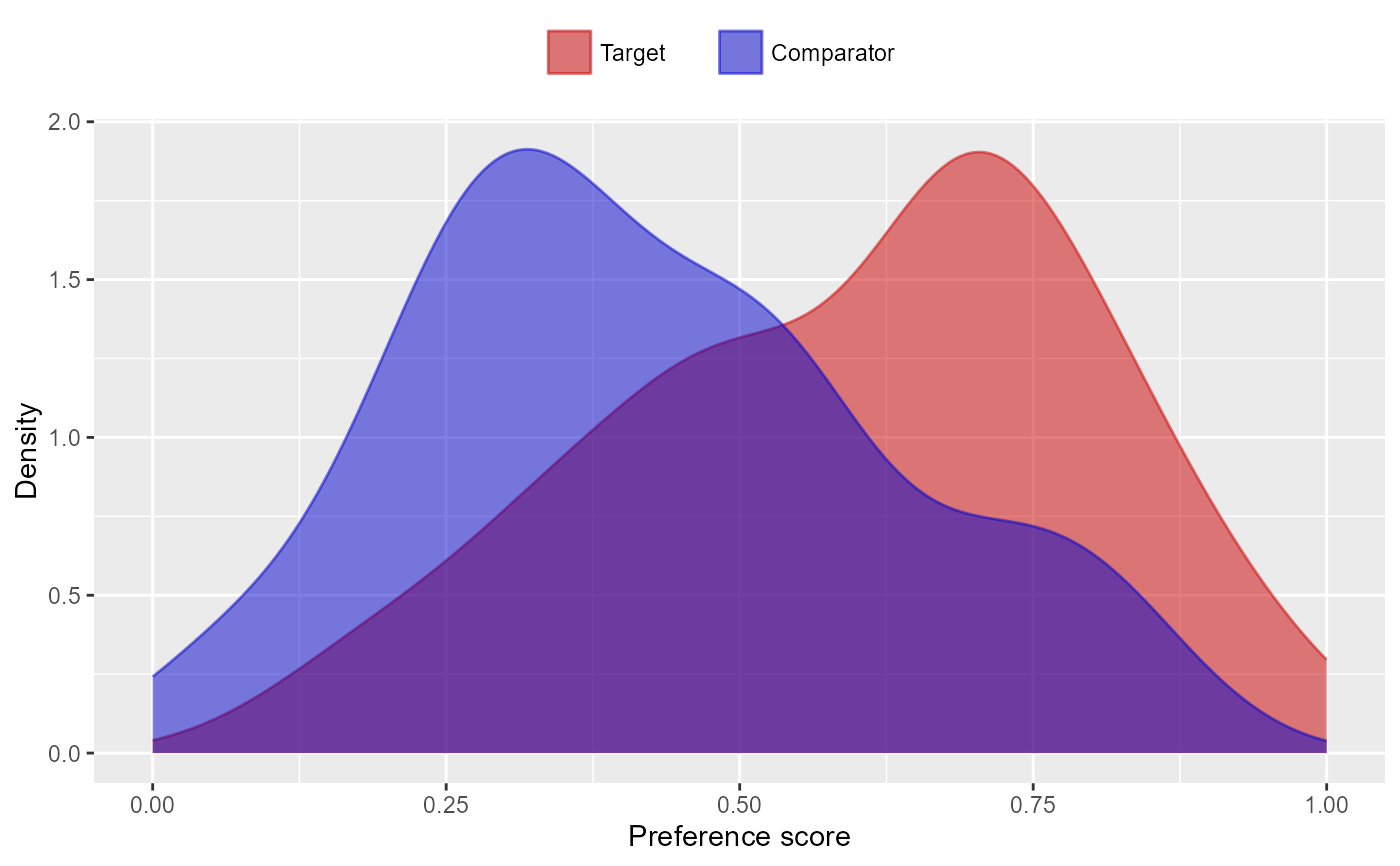Plots the propensity (or preference) score distribution.

plotPs(
data,
unfilteredData = NULL,
scale = "preference",
type = "density",
binWidth = 0.05,
targetLabel = "Target",
comparatorLabel = "Comparator",
showCountsLabel = FALSE,
showAucLabel = FALSE,
showEquiposeLabel = FALSE,
equipoiseBounds = c(0.3, 0.7),
unitOfAnalysis = "subjects",
title = NULL,
fileName = NULL
)

## Arguments

data

A data frame with at least the two columns described below

unfilteredData

To be used when computing preference scores on data from which subjects have already been removed, e.g. through trimming and/or matching. This data frame should have the same structure as data.

scale

The scale of the graph. Two scales are supported: scale = 'propensity' or scale = 'preference'. The preference score scale is defined by Walker et al (2013).

type

Type of plot. Four possible values: type = 'density' type = 'histogram', type = 'histogramCount', or type = 'histogramProportion'. 'histogram' defaults to 'histogramCount'.

binWidth

For histograms, the width of the bins

targetLabel

A label to us for the target cohort.

comparatorLabel

A label to us for the comparator cohort.

showCountsLabel

Show subject counts?

showAucLabel

Show the AUC?

showEquiposeLabel

Show the percentage of the population in equipoise?

equipoiseBounds

The bounds on the preference score to determine whether a subject is in equipoise.

unitOfAnalysis

The unit of analysis in the input data. Defaults to 'subjects'.

title

Optional: the main title for the plot.

fileName

Name of the file where the plot should be saved, for example 'plot.png'. See the function ggplot2::ggsave() for supported file formats.

## Value

A ggplot object. Use the ggplot2::ggsave() function to save to file in a different format.

## Details

The data frame should have a least the following two columns:

• treatment (integer): Column indicating whether the person is in the target (1) or comparator (0) group

• propensityScore (numeric): Propensity score

## Examples

treatment <- rep(0:1, each = 100)
propensityScore <- c(rnorm(100, mean = 0.4, sd = 0.25), rnorm(100, mean = 0.6, sd = 0.25))
data <- data.frame(treatment = treatment, propensityScore = propensityScore)
data <- data[data$propensityScore > 0 & data$propensityScore < 1, ]
plotPs(data)